# The Computer Vision Lab at GET

Department of Electrical Engineering
GET - Grundlagen der ElektroTechnik
Associated Member of the Heinz Nixdorf Institute (HNI)

# Gabor Filters

Gabor filters are bandpass filters which are used in image processing for feature extraction, texture analysis  , and stereo disparity estimation [9,10,13-15,24,30]. The impulse response of these filters is created by multiplying an Gaussian envelope function with a complex oscillation. Gabor   showed that these elementary functions minimize the space (time)-uncertainty product. By extending these functions to two dimensions it is possible to create filters which are selective for orientation   . Under certain conditions the phase of the response of Gabor filters is approximately linear. This property is exploited by stereo approaches which use the phase-difference of the left and right filter responses to estimate the disparity in the stereo images [9,10,13-15,24,30]. It was shown by several researchers that  the profile of simple-cell receptive fields in the mammalian cortex can by discribed by oriented two-dimensional Gabor functions [2,3,6,8,16-20,22,23,25-29].

Let x=[x1 x2]T be the image coordinates. The impulse response of a Gabor filter g(x) is then given by: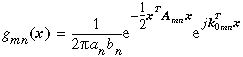Here, the matix A determines the bandwidth and the orientation selectivity of the filter.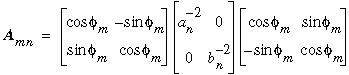If the modulation frequency vektor k0 is in the same direction  as one of the envelopes axes, with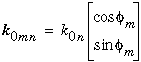then the real part and the imaginary part of the filter's impulse response are looking as follows: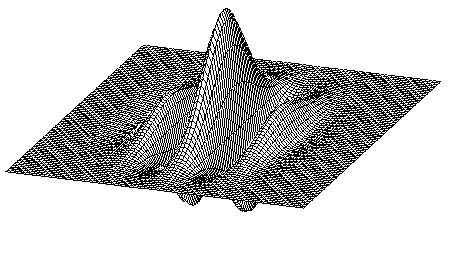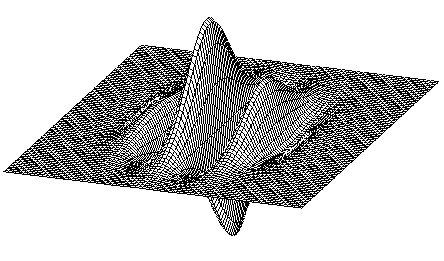Real part and imaginary part of the impulse response of a Gabor filter

The transfer function G(k) of a Gabor filter (Fourier transform of the impulse response) is given by: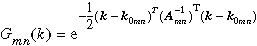where k = [k1 k2]T is the spatial frequency. In oder to establish a multi-resolution strategy the image can be filtered with a set of  N Gabor filters with different bandwidths and modulation frequencies. If the modulation frequencies are given by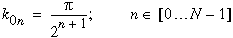and the relative bandwidth is choosen to be constant for all filters the image is decomposed into octaves.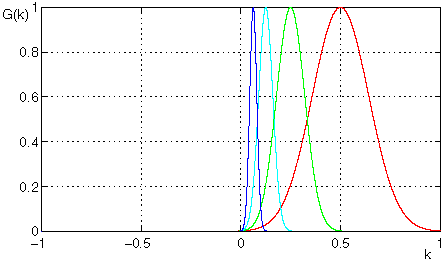Magnitude of a Gabor filter set for N=4 in direction of the modulation frequency

In the figure shown above the transfer functions of the filters are choosen to overlap at a value of 0.5. Under this condition the DC component in the images and the frequency components lying above the frequency twice as large as the modulation freqency are damped by at least -54dB. Thus the filter results can be sub-sampled by a factor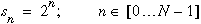with neglectable aliasing effects.  Furthermore the images can be decomposed into M channels of different orientations by using filters tuned to different angles: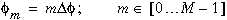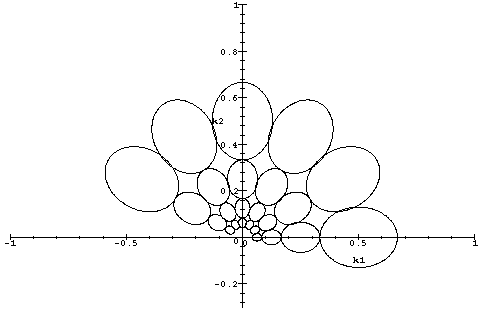Half-value  plot of the Gabor filters in the frequency plane tuned to different frequencies  and orientations (30 degree resolution)

The  responses of the filters lying in the lower frequency half-plane need not to be calculated explicitely, because they can be generated by the  complex-conjugation of  the filter response  lying on the other side of the origin. The following images show the original Lenna picture and Gabor filter results for different sampling factors. The size of the input image is choosen such that the filter results have always the same size after subsampling. This strategy leads to a multy resolution architecture which is also called Gabor  pyramid. In the best resolution channel it is possible to extract fine image structures of a small image region whereas in the lower resolution levels coarse image structures can be extracted over large regions. This can be exploited in coarse-to-fine strategies [4,5]. On the left side of the following pictures the original input (sub) images and the superposed filter results for all orientations are shown. On the right side the subsampled filter result is shown for each orientation.

s = 4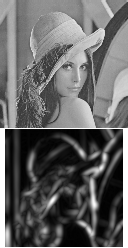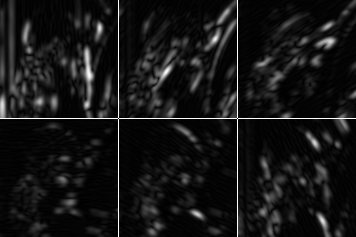s = 2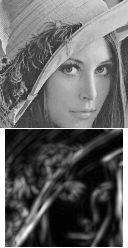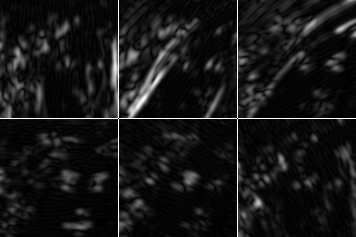s = 1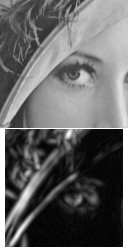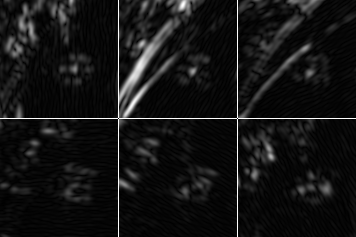Subimages of the Lenna-picture and magnitudes of the corresponding filter results

We use a set of Gabor filters as a preprocessing step for estimation of stereo disparity and extraction of oriented image features in the DEMON project

Last change: 17.11.1998 (R.Trapp)